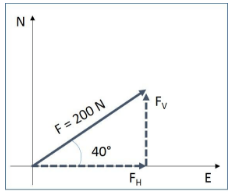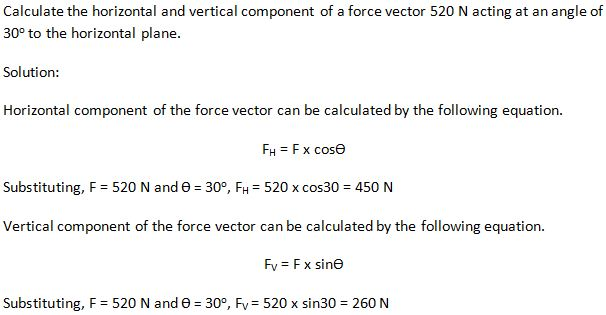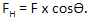# Resolving Vectors

## Introduction

A quantity with magnitude and direction is defined as a vector quantity. The weight of an object, velocity, and acceleration of a vehicle and the force acting on a bridge are some examples of vector quantity. All vector quantities can be split, or resolved, into two components: Horizontal components and vertical components. The combined effect of the components is the same as the original vector. Resolving vectors into horizontal and vertical components is used in the addition and subtraction of vectors and finding the resultant of multiple vectors.

How to Resolve a Vector

Consider the force illustrated in Figure 1. The force vector at a 400 angle can be split, or resolved, into two vectors or components as illustrated in Figure 2. The following equations shall be used to resolve any vector quantity.Figure 1: Vector IllustrationFigure 2: Resolution of Vector

A. Example: 1B. Example: 2C. Summary

• Any vector quantity can be split into horizontal and vertical components. The combined effect of the horizontal and vertical components of the vector quantity is the same as the original vector.
• Horizontal components of a vector quantity can be calculated by the equation• Vertical components of a vector quantity can be calculated by the equation,